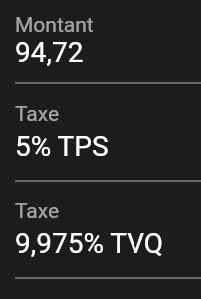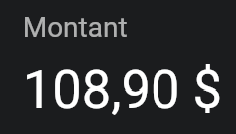# Tax calculations: slight rounding errors | v5.3.81

Hi,

I noticed what appears to be some rounding errors when adding taxes to expenses. It’s hard to tell what number exactly does not gets calculated right, because the taxes (two of them) get bundled together in the admin panel and in the reports. I think it’s related to “document rounding” vs “line item rounding”, as discussed here:
Tax calculations differ - Discourse (invoiceninja.com)

Taxes should be rounded one by one instead of together at the end - at least that’s how it works in Canada, and I’m pretty sure that’s how it works elsewhere with multiple added taxes too. Maybe there should be an option to set rounding per-tax?

Below is an example of a miscalculation in IN - well, not a miscalculation in itself, but according to the way the taxes should be calculated. First, here is the amount on which the taxes are applied:0.05 * 94.72 = 4.736 → 4.74 rounded
0.09975 * 94.72 = 9.44832 → 9.45 rounded

If we add the taxes and the taxable amount, we get 94.72 + 4.74 + 9.45 = 108.91

However, here is what IN gives me:It’s 1 penny short. But it makes sense if the calculation was 94.72 + 4.736 + 9.44832 = 108.90432 → 108.90

So all in all, if we could get a way to change the way rounding is done (per tax vs per document), that would fix this.

Thanks!

@charles

Does the PDF show correctly, or do both the UI and PDF show the same calculated amount?

I believe this is an expense issue so the rounding issue is in the admin portal.

We’ll make sure this is corrected in the next release.

Hi @david, as @hillel said, it’s related to expenses calculations in the admin portal; I haven’t tried adding those expenses to an invoice so far (as I don’t usually bill my expenses this way).

I’ll update to .84 and check if the issue has been corrected.

Thanks!